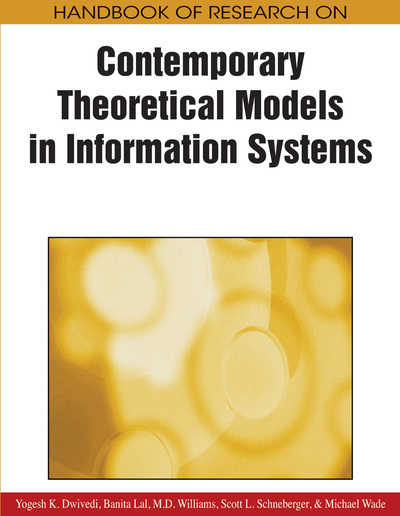# Theory Development in Information Systems Research Using Structural Equation Modeling: Evaluation and Recommendations

Nicholas Roberts (Clemson University, USA) and Varun Grover (Clemson University, USA)
DOI: 10.4018/978-1-60566-659-4.ch005
Available
\$37.50
No Current Special Offers

## Abstract

Structural equation modeling (SEM) techniques have significant potential for assessing and modifying theoretical models. There have been 171 applications of SEM in IS research, published in major journals, most of which have been after 1994. Despite SEM’s surging popularity in the IS field, it remains a complex tool that is often mechanically used but difficult to effectively apply. The purpose of this study is to review previous applications of SEM in IS research and to recommend guidelines to enhance the use of SEM to facilitate theory development. The authors review and evaluate SEM applications, both component-based (e.g., PLS) and covariance-based (e.g., LISREL), according to prescribed criteria. Areas of improvement are suggested which can assist application of this powerful technique in IS theory development.
Chapter Preview
Top

## Overview Of Structural Equation Modeling

To provide a basis for subsequent discussion, we present a brief overview of SEM. SEM is a technique used to specify, estimate, and evaluate models of linear relationships among a set of observed variables in terms of a generally smaller number of unobserved variables. Figure 1 depicts a basic latent variable model. A circle is used to represent each of the four latent variables, and the boxes represent associated manifest or indicator variables. The relationships between the latent variables and their indicators are often referred to as a “measurement” model, in that it represents an assumed process in which an underlying construct determines or causes behavior that is reflected in measured indicator variables.

Within this context, it is important to note that the arrows go from the circles to the boxes, which is consistent with the process noted above. Thus, each factor serves as an independent variable in the measurement model, and the indicator variables serve as the dependent variables. Each indicator is also potentially influenced by a second independent variable in the form of measurement error, and its influence is represented as a cause of the indicator variable through the use of a second arrow leading to each of the indicators. Finally, the model shown in Figure 1 includes correlations (double-headed arrows) among the three exogenous constructs (LV1–LV3) and regression-like structural parameters linking exogenous and endogenous constructs (e.g., LV3, LV4). The model also acknowledges that there is unexplained variance in the endogenous latent variable. The part of the overall model that proposes relationships among the latent variables is often referred to as the structural model.

## Key Terms in this Chapter

Construct Validity: The extent to which a given test is an effective measure of a theoretical construct

Structural Equation Modeling: Multivariate technique combining aspects of multiple regression (examining dependence relationships) and factor analysis (representing unmeasured concepts with multiple variables) to estimate a series of interrelated dependence relationships simultaneously

Measurement Model: Sub-model in structural equation modeling that specifies the indicators for each construct and assesses the reliability of each construct for estimating the causal relationships

Latent Variable: Research construct that is not observable or measured directly, but is measured indirectly through observable variables that reflect or form the construct

Factor Analysis: A statistical approach that can be used to analyze interrelationships among a large number of variables and to explain these variables in terms of their common underlying dimensions (factor)

Reliability: Extent to which a variable or set of variables is consistent in what it is intended to measure

Theory: A statement of relations among concepts within a set of boundary assumptions and constraints

Structural Model: Linkages between research constructs (or variables) that express the underlying structure of the phenomenon under investigation

## Complete Chapter List

Search this Book:
Reset